# MISSION BANKING 2023 Quantitative Aptitude Quiz- 27

## MISSION BANKING 2023 Quantitative Aptitude Quiz

Quantitative aptitude measures a candidate’s numerical proficiency and problem-solving abilities. It is the most important section of almost all competitive exams. Candidates are often stymied by the complexity of Quantitative Aptitude Questions but if they practice more and more questions it will become quite easy. So, here we are providing you with the MISSION BANKING 2023 Quantitative Aptitude Quiz to enhance your preparation for your upcoming examination. Questions given in this MISSION BANKING 2023 Quantitative Aptitude Quiz are based on the most recent and the latest exam pattern. A detailed explanation for each question will be given in this MISSION BANKING 2023 Quantitative Aptitude Quiz. This MISSION BANKING 2023 Quantitative Aptitude Quiz is entirely free of charge. This MISSION BANKING 2023 Quantitative Aptitude Quiz will assist aspirants in achieving a good score in their upcoming examinations.

Direction (1-5): What will come at the place of question (?) marks:

1. 884, 644,     452,     308,     ?,     164

(a) 210

(b) 202

(c) 198

(d) 212

(e) 2161. 557, 605,     658,     721,     799,     ?

(a) 897

(b) 887

(c) 817

(d) 878

(e) 899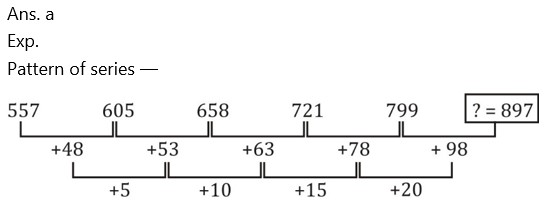1. 996, 500,     504,     762,     1532,     ?

(a) 3850

(b) 3844

(c) 3848

(d) 3840

(e) 3860

Ans. d

Exp.

Pattern of series —

996 × 0.5 + 2 = 500

500 × 1 + 4 = 504

504 × 1.5 + 6 = 762

762 × 2 + 8 = 1532

? = 1532 × 2.5 + 10 = 3840

1. 3067, 1736,     1007,     664,     539 ,     ?

(a) 506

(b) 512

(c) 510

(d) 508

(e) 5241. 128, 130,     263,     793,     ?,     15891

(a) 3177

(b) 3179

(c) 3175

(d) 3178

(e) 3198

Ans. a

Exp.

Pattern of series —

128 × 1 + 2 = 130

130 × 2 + 3 = 263

263 × 3 + 4 = 793

? = 793 × 4 + 5 = 3177

3177 × 5 + 6 = 15891

Directions (6-10): Each of the questions below consists of a question and two statements numbered I and II given below it. You have to decide whether the data provided in the statements are sufficient to answer the question. Read both the statements and give answer

1. What is the speed of a boat in still water ?

I. The boat covers a distance of 160 km in 8 hours while running upstream.

II. It covers the same distance in 4 hours while running downstream.

(a) if the data in statement I alone are sufficient to answer the question, while the data in statement II alone are not sufficient to answer the question.

(b) if the data in statement II alone are sufficient to answer the question, while the data in statement I alone are not sufficient to answer the question.

(c) if the data either in statement I alone or in statement II alone are sufficient to answer the question.

(d) if the data in both statement I and II together are not sufficient to answer the question.

(e) if the data in both statement I and II together are necessary to answer the question.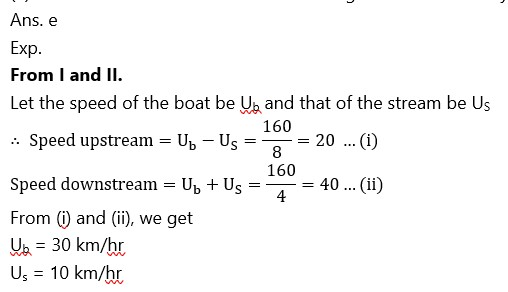1. How many boys are there in the class ?

I. The number of girls is 25% of the number of boys.

II. The ratio of the number of boys to that of girls is 5 : 7.

(a) if the data in statement I alone are sufficient to answer the question, while the data in statement II alone are not sufficient to answer the question.

(b) if the data in statement II alone are sufficient to answer the question, while the data in statement I alone are not sufficient to answer the question.

(c) if the data either in statement I alone or in statement II alone are sufficient to answer the question.

(d) if the data in both statement I and II together are not sufficient to answer the question.

(e) if the data in both statement I and II together are necessary to answer the question.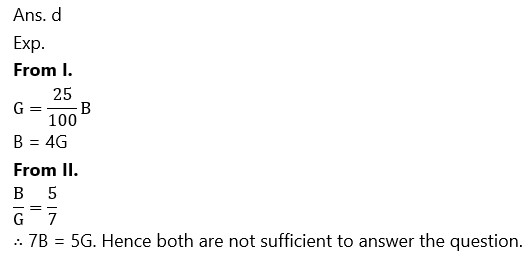1. What is the value of a two-digit number ?

I. The sum of the digits of the number is 13.

II. The digit at the tens place is 160% of the digit at the units place.

(a) if the data in statement I alone are sufficient to answer the question, while the data in statement II alone are not sufficient to answer the question.

(b) if the data in statement II alone are sufficient to answer the question, while the data in statement I alone are not sufficient to answer the question.

(c) if the data either in statement I alone or in statement II alone are sufficient to answer the question.

(d) if the data in both statement I and II together are not sufficient to answer the question.

(e) if the data in both statement I and II together are necessary to answer the question.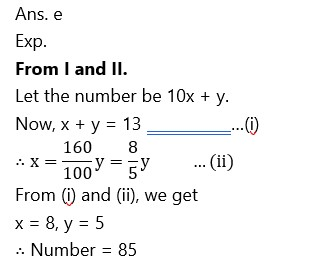1. What is the area of a square ?

I. The diagonal of the square is 82 m.

II. The perimeter of the square is 32 m.

(a) if the data in statement I alone are sufficient to answer the question, while the data in statement II alone are not sufficient to answer the question.

(b) if the data in statement II alone are sufficient to answer the question, while the data in statement I alone are not sufficient to answer the question.

(c) if the data either in statement I alone or in statement II alone are sufficient to answer the question.

(d) if the data in both statement I and II together are not sufficient to answer the question.

(e) if the data in both statement I and II together are necessary to answer the question.1. What is the speed of a train ?

I. The train crosses a pole in 16 seconds.

II. The train crosses a platform of equal length in 54 seconds.

(a) if the data in statement I alone are sufficient to answer the question, while the data in statement II alone are not sufficient to answer the question.

(b) if the data in statement II alone are sufficient to answer the question, while the data in statement I alone are not sufficient to answer the question.

(c) if the data either in statement I alone or in statement II alone are sufficient to answer the question.

(d) if the data in both statement I and II together are not sufficient to answer the question.

(e) if the data in both statement I and II together are necessary to answer the question.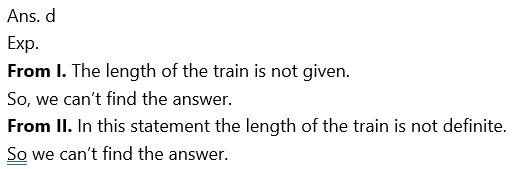### 2023 Preparation Kit PDF

#### Most important PDF’s for Bank, SSC, Railway and Other Government Exam : Download PDF Now

AATMA-NIRBHAR Series- Static GK/Awareness Practice Ebook PDF Get PDF here
The Banking Awareness 500 MCQs E-book| Bilingual (Hindi + English) Get PDF here
AATMA-NIRBHAR Series- Banking Awareness Practice Ebook PDF Get PDF here
Computer Awareness Capsule 2.O Get PDF here
AATMA-NIRBHAR Series Quantitative Aptitude Topic-Wise PDF 2020 Get PDF here
Memory Based Puzzle E-book | 2016-19 Exams Covered Get PDF here
Caselet Data Interpretation 200 Questions Get PDF here
Puzzle & Seating Arrangement E-Book for BANK PO MAINS (Vol-1) Get PDF here
ARITHMETIC DATA INTERPRETATION 2.O E-book Get PDF here

3